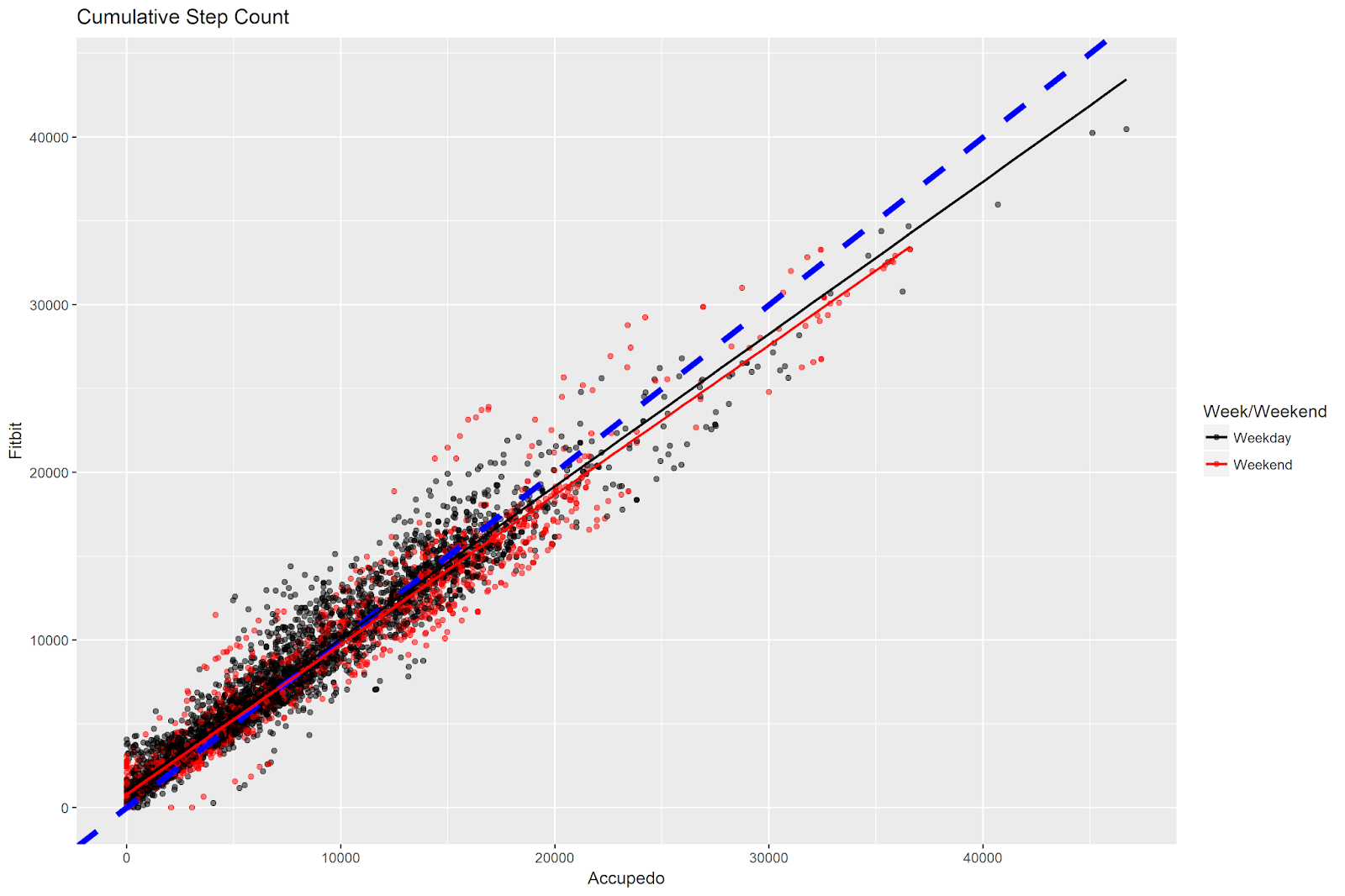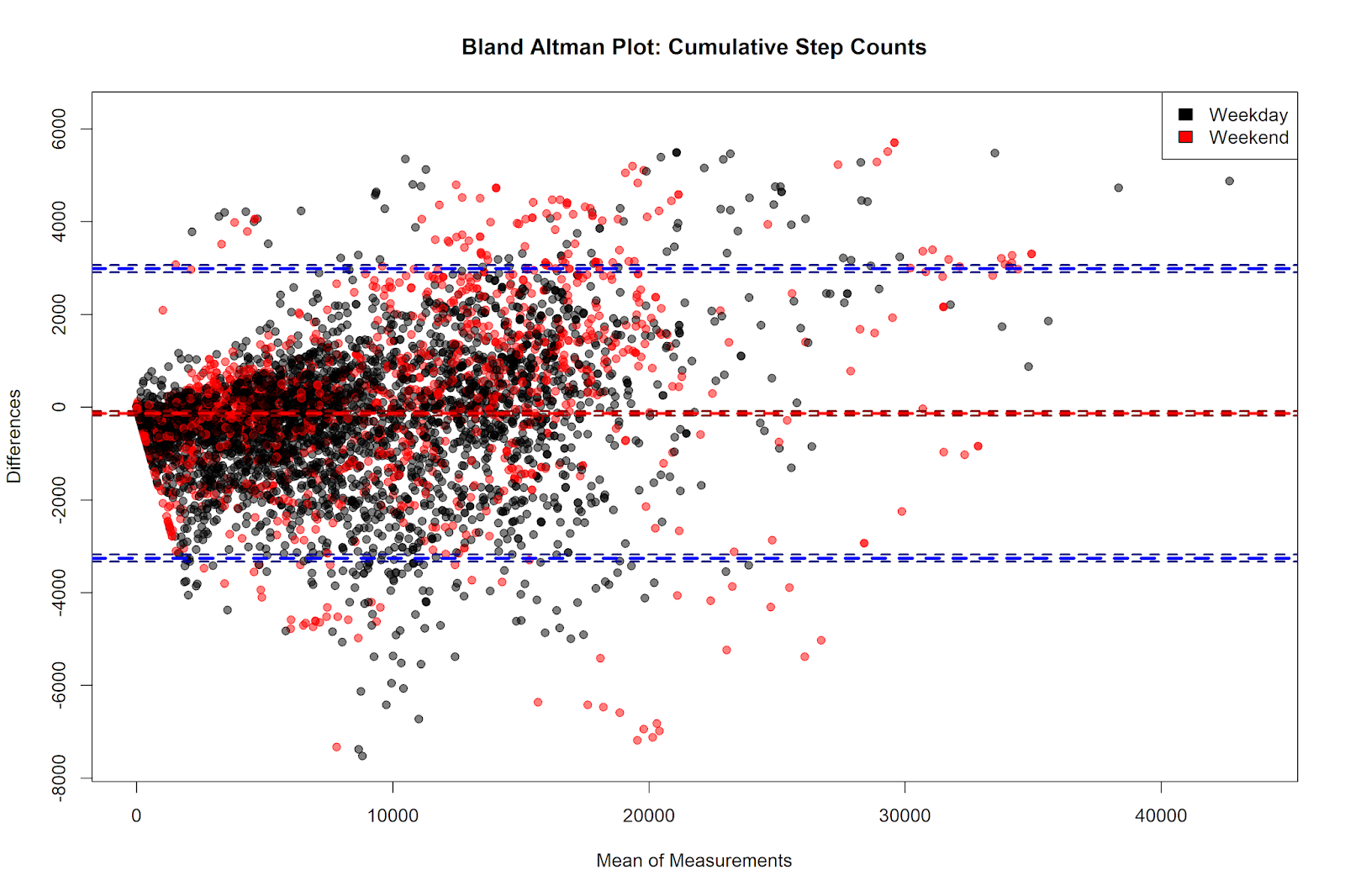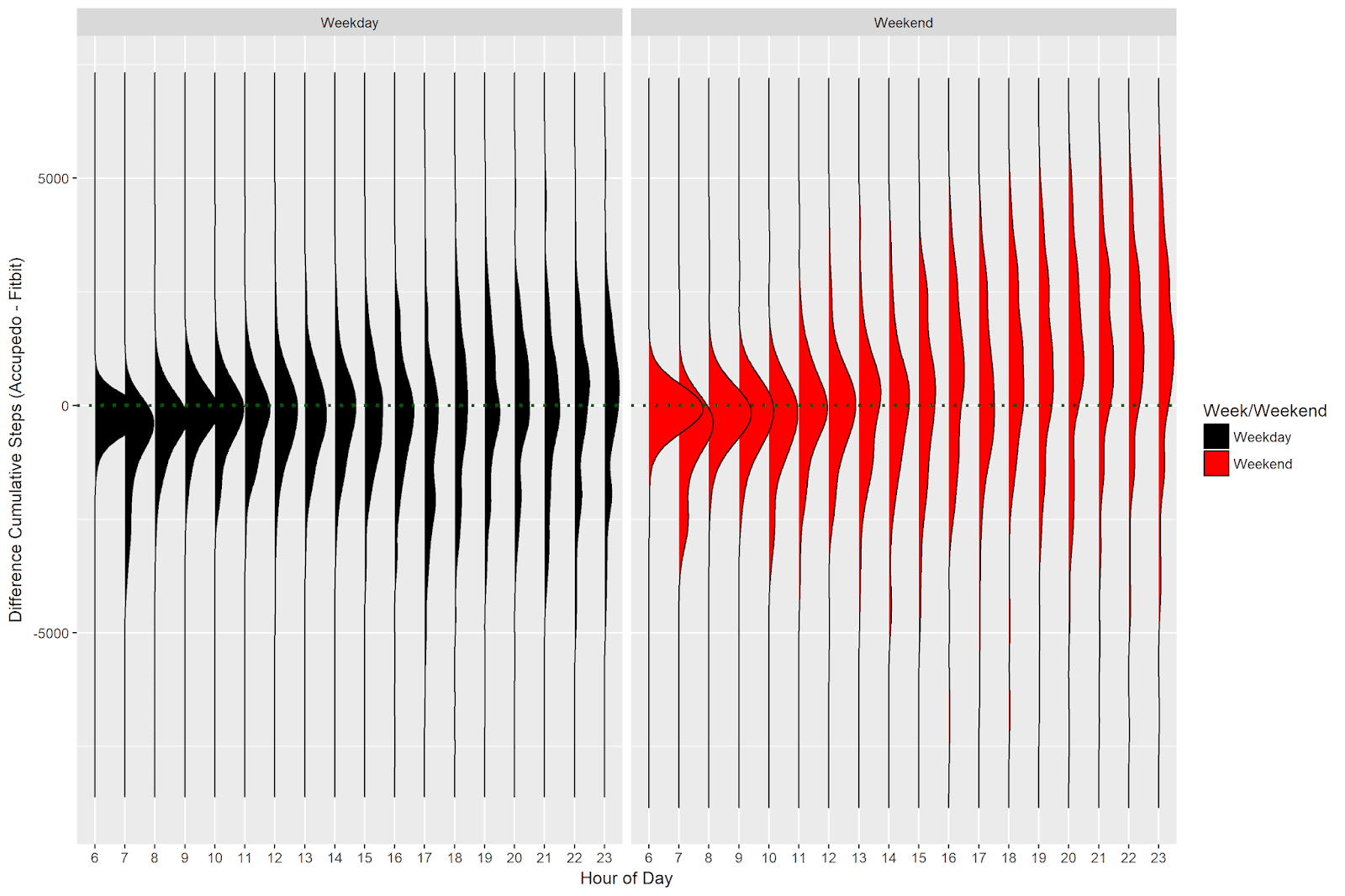# Accupedo vs. Fitbit Part 2: Convergent Validity of Cumulative Step Counts with R

2019, Nov 25

This post is a continuation of the previous post on this blog. Last time, we analyzed hourly step count data from the Accupedo app on my phone, and from the Fitbit I wear on my wrist. This time, we will analyze the cumulative step count measurements taken from Accupedo and Fitbit. This metric is arguably more interesting from a user perspective. After all, when you check your steps on the respective devices, you always see the cumulative step count - e.g. the number of steps that you have taken thus far that day. Do these devices give similar readings of cumulative step counts? When are the two measurements more likely to agree or disagree with one another?

## The Data

The data come from two sources: the Accupedo app on my phone and from the Fibit (model Alta HR) that I wear on my wrist. Both data sources are accessible (with a little work) via R: you can see my write up of how to access data from Accupedo here and my post on how to access data from Fitbit here.

I got the Fitbit in March 2018, and the data from both devices were extracted in mid-December 2018. I was able to match 273 days for which I had step counts for both Accupedo and Fitbit. The data contain the hourly and cumulative steps for the hours from 6 AM to 11 PM. In total, the dataset contains 4,914 observations of hourly step counts for the 273 days for which we have data (e.g. 18 observations per day).

You can find the data and all the code from this blog post on Github here.

The head of the dataset (named merged_data) looks like this:

date daily_total_apedo hour hourly_steps_apedo cumulative_daily_steps_apedo daily_total_fbit hourly_steps_fbit cumulative_daily_steps_fbit dow week_weekend hour_diff_apedo_fbit
2018-03-20 16740 6 0 0 15562 281 281 Tue Weekday -281
2018-03-20 16740 7 977 977 15562 1034 1315 Tue Weekday -57
2018-03-20 16740 8 341 1318 15562 1605 2920 Tue Weekday -1264
2018-03-20 16740 9 1741 3059 15562 223 3143 Tue Weekday 1518
2018-03-20 16740 10 223 3282 15562 287 3430 Tue Weekday -64
2018-03-20 16740 11 226 3508 15562 188 3618 Tue Weekday 38
2018-03-20 16740 12 283 3791 15562 1124 4742 Tue Weekday -841
2018-03-20 16740 13 1587 5378 15562 525 5267 Tue Weekday 1062
2018-03-20 16740 14 431 5809 15562 372 5639 Tue Weekday 59
2018-03-20 16740 15 624 6433 15562 392 6031 Tue Weekday 232

## Cumulative Step Counts

### Correspondence Plot

In this post, we will explore the cumulative step counts. We can make a scatterplot showing the correspondence between the cumulative step counts (coloring the points by type of day - week vs. weekend), and compute their correlation, using the following code:

Which returns the following plot:In this plot, the points are colored by weekday/weekend, and separate regression lines are drawn for each type of day. The dashed blue line is the identity line - if both Accupedo and Fitbit recorded the same number of cumulative steps each hour, all of the points would lie on this line.

Especially in comparison to the hourly plot, the correspondence is good! Both regression lines are very close to the identity line, suggesting a high degree of agreement between the cumulative step counts on both weekdays and weekends.

There is a slight over-representation of red points below the identity line, and a slight over-representation of black points above the identity line. This indicates that we see more weekend days (red points) where Accupedo has higher cumulative step measurements than Fitbit, and more weekday days (black points) where Fitbit has higher cumulative step measurements than Accupedo.

The code above computes the correlation between the cumulative step count measurements for the two devices. This correlation is .97, which indicates extremely high agreement. Keep in mind that the correlation between the hourly step counts was only .52. In comparison, the cumulative step counts match much more closely with one another!

### Bland Altman Plot

Another way of examining the correspondence between two measurements is the Bland-Altman plot. The Bland-Altman plot displays the mean of the measurements on the x-axis, and the difference between the measurements on the y-axis. A horizontal line (in red in the plot blow) is drawn on the plot to indicate the mean difference between the measurements. In addition, two lines (in blue in the plot below) are drawn at +/- 1.96 standard deviations above and below the mean difference, respectively.

We will use the excellent BlandAltmanLeh package in R to make the Bland-Altman plot. Note that it takes some additional work to get the plot to have the same color scheme as our above correlation plot, with separate colors for weekdays and weekends, and transparency in the points.

Which gives us the following plot:There are a couple of things to notice here. The first is that the mean of the difference of the cumulative step counts - shown on the y axis - lies below zero (the exact value is -135.94, as we’ll see below). The y axis shows the value of the Accupdeo steps minus the Fitbit steps, and so the negative difference indicates that Accupedo gives lower cumulative step counts than Fitbit on average. However, the size of this hourly difference (in comparison to the range of differences across the data) is small.

Take a look at the points that sit above and below the blue lines - these are the points that are more than 1.96 standard deviations above or below the global average. We see a slightly higher concentration of red points above 1.96 standard deviations from the mean. Conversely, we see a slightly higher concentration of black points below 1.96 standard deviations from the mean. This indicates that we see more observations on weekends for which Accupedo gives a much higher cumulative step count than Fitbit. We see more observations on weekdays for which Fitbit gives a much higher cumulative step count than Accupedo. This was also one of the conclusions from the scatterplot above!

### Testing the Statistical Significance of the Cumulative Differences

A corresponding statistical analysis that often accompanies the Bland-Altman plot is a one-sided t-test, comparing the mean difference of the measurements against zero (null hypothesis: the mean difference between the measurements is equal to zero). The mean of the differences is -135.94 and the standard deviation is 1592.76.

This test indicates that the difference between the cumulative step counts for Accupedo and Fitbit is statistically significant, t(4913) = -5.98, p < .001.

Let’s explore the effect size of this comparison. Effect sizes give a measure of the magnitude of an observed difference. There are many such measures, but we can compute Cohen’s D from the values we have above. Cohen’s D gives the size of a difference scaled to the standard deviation of that difference. Here, we simply take the mean difference score (-135.94) and divide it by the standard deviation of that score (1592.76), which gives us a Cohen’s D value of -.09. In other words, the cumulative step count measurements from Accupedo and Fitbit differ by less than 1/10th of a standard deviation. According to the standards laid out by Cohen (1988), the effect size is very small.

The global conclusion is that the cumulative steps recorded by Fitbit are systematically higher than the cumulative steps recorded by Accupedo, but that the size of this difference is very small!

## Differences in Cumulative Step Counts Across the Day

The above plots mix data across all hours of the day in order to examine the global correspondence between cumulative step counts. In the next analysis we will visualize the difference in the cumulative step count measurements across the hours of the day, to see if there were any systematic differences within certain times of the day.

To make this plot, we’ll use the excellent ggridges package, visualizing the density distributions of step count differences separately for each hour of the day, with separate panels for weekdays and weekends.

Which gives us the following plot:Interestingly, there do appear to be differences in the distributions of the differences in cumulative step counts across the hours of the day. The green horizontal dotted line is drawn at zero, the point at which there are no differences in cumulative step counts. The y-axis shows the result of the Accupedo steps minus the Fitbit steps; data below the line indicate higher cumulative counts for Fitbit, while data above the line indicate higher cumulative counts for Accupedo.

On both weekdays and weekends, the day starts with a distribution which has more observations for which the Fibit cumulative counts are higher (as the peak of the distributions lie below the dotted line). This is likely due to the fact that any movement that occurs at night is picked up by the Fitbit (which is on my wrist all night) and not by Accupedo (because I don’t have my phone in hand all night). So we start the day with a higher cumulative step count with Fitbit than we do with Accupedo.

The distribution slowly starts shifting upward throughout the day. At around noon on weekdays and at around 11 AM on the weekends, the distribution is more-or-less centered at zero, indicating that the average cumulative step counts are the same at this point in the day.

The further along we get during the day, the distributions become much flatter and less centered around zero. The global trend at the end of the day, especially pronounced on the weekends, is to have a relatively flat distribution with a greater number of observations for which Accupedo has higher cumulative step counts than Fitbit.

### Testing the Difference in Cumulative Step Counts Between Weekdays vs. Weekends

We saw above that the overall difference between the cumulative counts for Accupedo and Fitbit was -135.94, indicating that across all observations, the Fitbit cumulative counts were 136 steps higher. The distribution plot above suggests a nuance, in that the relative difference appears to reverse on the weekends. (Because we have many more weekday observations then weekend observations, the global average is heavily weighted by the weekdays, in which Fitbit gives higher step counts).

Let’s examine whether the cumulative step count differences are different on weekdays vs. weekends. We can do this in a very simple way via a linear model, in which we predict the cumulative difference score using the categorical variable of week/weekend. We do this via the regression specified below. We then ask for a summary of the results of the model.

The code to run the regression looks like this:

And returns the following regression output:

 Dependent variable: cumul_diff_apedo_fbit week_weekendWeekend 447.89*** (49.89) Constant -263.91*** (26.67) Observations 4,914 R2 0.02 Adjusted R2 0.02 Residual Std. Error 1,580.01 (df = 4912) F Statistic 80.58*** (df = 1; 4912) Note: *p<0.1; **p<0.05; ***p<0.01

The intercept (or “constant” as it’s described in the table above) is -263.91, indicating that on weekdays (when the dummy variable for week_weekend is 0), Fitbit yields an average cumulative step count that is 263.91 steps higher than that for Accupedo. However, on weekends (when the dummy variable for week_weekend is 1), the average difference between the cumulative step counts is 183.98 (e.g. 447.89 + -263.91) steps higher for Accupedo. Note that the R2 value is very small - most of the variation in cumulative step count differences is not accounted for by the week/weekend variable!

In sum, the nuanced conclusion is this: the cumulative step counts are on average higher for Fitibit (vs. Accupedo), but on weekends the pattern reverses, such that on average Accupedo gives higher cumulative step counts as compared to Fitbit. In both cases, the differences are small (less than 300 steps in either direction).

## Summary and Conclusion

In this post, we examined the convergent validity of cumulative step counts from the Accupedo app on my phone and the Fitbit I wear on my wrist. The correlation between these two devices’ records was .97, indicating a very strong relationship between the two measurements of cumulative step counts.

We then constructed a Bland-Altman plot to compare the two measurements. This plot revealed that on average Fitbit’s cumulative step count was 135.94 steps higher than Accupedo’s. While statistically significant, the size of this difference was equivalent to 1/10th of a standard deviation; in other words - a very small effect.

Both the correpsondence plot and the Bland-Altman plot showed a number of observations for which Fitbit had higher cumulative step counts during weekdays, and a number of observations for which Accupedo had higher cumulative step counts during weekends.

When examining the distributions of step count differences across hours of the day, we got some more insight into this pattern. On both weekdays and weekends, in the first hours of the day, Fitbit consistently records higher cumulative step counts than Accupedo, most likely because Fitbit counts night time activitity (because it’s on my wrist), whereas Accupedo does not (because it’s on my phone). Throughout the day, however, the distribution of the differences shifts towards higher cumulative step counts for Accupedo. This difference is especially pronounced on the weekend.

Indeed, our regression analysis of the cumulative differences across weekdays and weekends indicated a reveral of the global pattern depending upon the type of day. During the weekdays, the pattern matches the overall trend - Fitbit yields slightly higher cumulative step counts than does Accupedo. However, on the weekend, the pattern reverses and the average cumulative step counts are higher for Accupedo. In both cases, the sizes of the differences between the devices is small.

#### Why does the distribution shift from higher counts for Fitbit to higher counts for Accupdeo throughout the day?

The days start out with a globally higher cumulative count for Fitbit, likely because it picks up night time activity that Accupedo does not. As we saw in the previous post, however, there is a tendency for Accupedo to give greater hourly step counts than Fitbit. As the day progresses, these small hourly differences seem to accumulate, shifting the balance in cumulative step counts by the day’s end.

#### Why is this difference more pronounced on the weekends?

I’m not quite sure about this. I’ve seen in previous analyses of my step count data that my walking patterns tend to be quite different on weekdays and the weekends. My guess is that the observed differences have to do with the types of walking I do. During the week, I tend to walk shorter distances in any one go, whereas on the weekend I tend to do longer walks throughout the day. Importantly, during the time that these data were recorded, I was more often pushing a stroller on the weekend (vs. weekdays). I’ve noticed that, because my wrist is continually horizontal when pushing a stroller, Fitbit counts fewer steps than does Accupedo (which is in my pocket). Perhaps that has something to do with this difference between weekdays and weekends, but it’s more of a guess than a data-driven conclusion.

## In Sum: Comparing Convergent Validity Between Hourly and Cumulative Step Counts

#### Hourly

The correspondence between the hourly step counts is not large (correlation of only .52), but there is no systematic difference in over or under-counting between the devices. Sometimes Accupedo counts more steps per hour, and sometimes Fitbit counts more steps per hour. There appear to be no systematic differences in hourly step counts during the weekday vs. the weekend.

#### Cumulative

In contrast, the correspondence between the cumulative step counts is substantial (correlation of .97). The devices’ measurements match quite closely, but there is a small but reliable difference in the cumulative step count measurements between devices, with Fitbit counting 135.94 more cumulative steps on average, compared to Accupedo. Examining the difference in cumulative step counts across the hours of the day shows that Fitbit has higher cumulative counts in the morning, but that this difference shifts towards Accupedo throughout the day. On the weekends, the global pattern reverses, with Accupedo yielding higher average cumulative step counts than Fitbit. In both cases, the difference in cumulative step counts between the devices is small.

Coming Up Next

In the next post, we will turn to a difference data source: detailed records of my phone usage. We will use data munging and visualization to see how and when my phone usage patterns differ throughout the day.

Stay tuned!